# Transformations What are transformations 1 2 3 4

• Slides: 19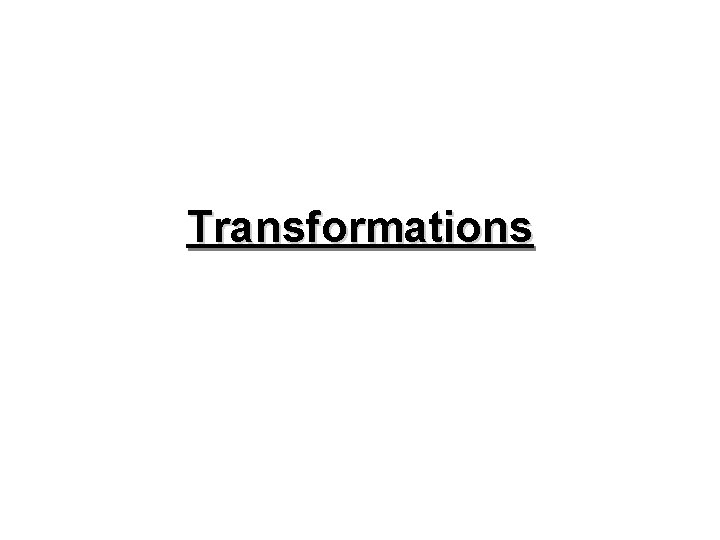TransformationsWhat are transformations? • • 1. 2. 3. 4. Any movement of a shape is called a transformation There are 4 types Reflection Translation Rotation Enlargement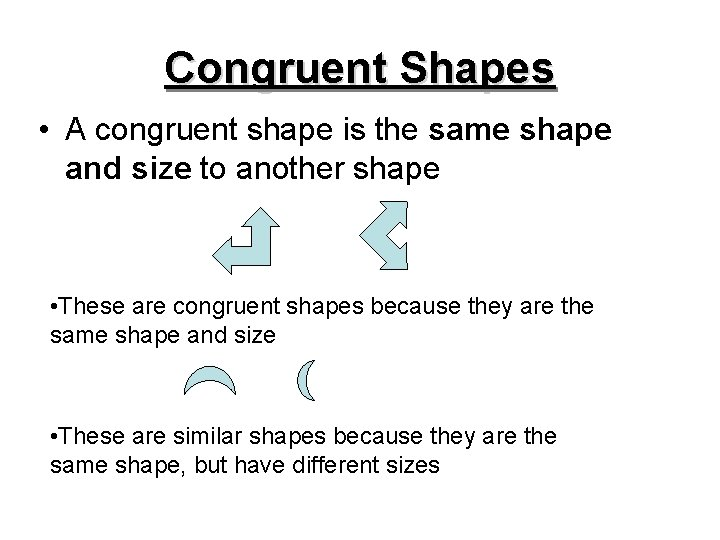Congruent Shapes • A congruent shape is the same shape and size to another shape • These are congruent shapes because they are the same shape and size • These are similar shapes because they are the same shape, but have different sizes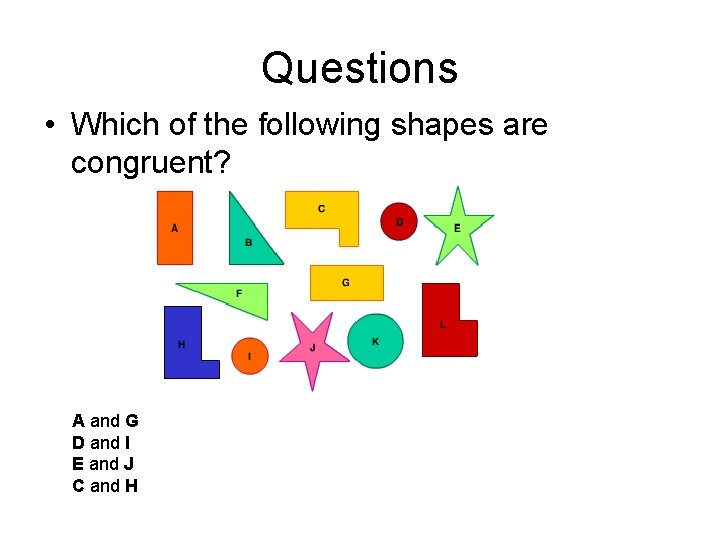Questions • Which of the following shapes are congruent? A and G D and I E and J C and H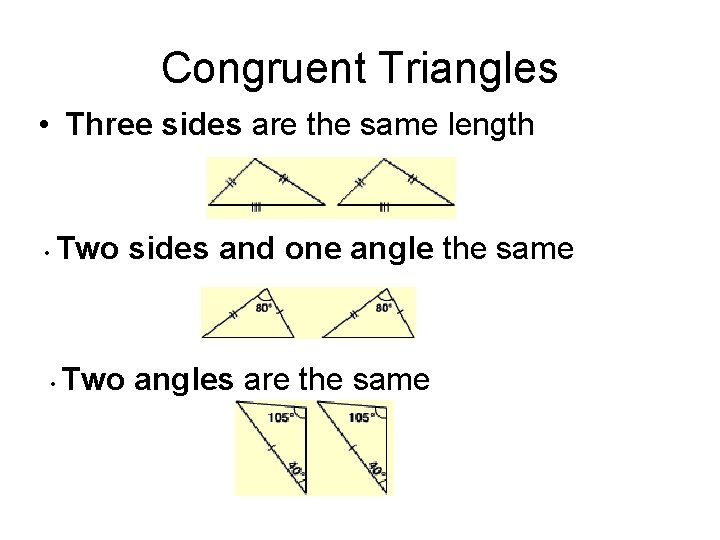Congruent Triangles • Three sides are the same length • Two sides and one angle the same • Two angles are the same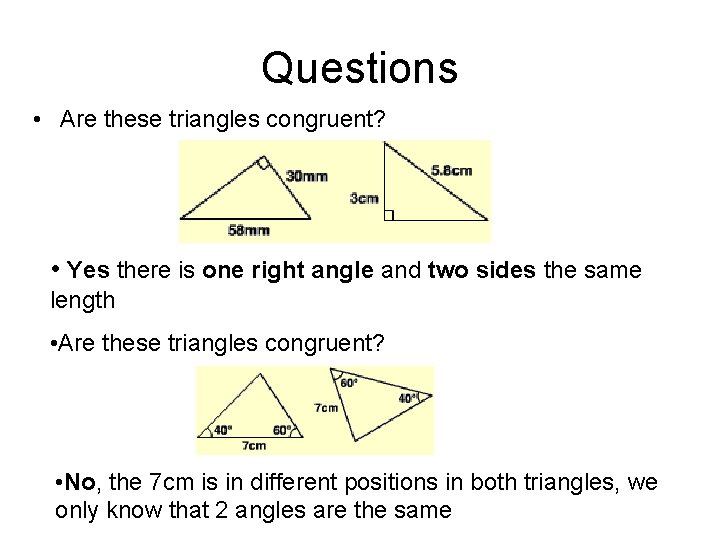Questions • Are these triangles congruent? • Yes there is one right angle and two sides the same length • Are these triangles congruent? • No, the 7 cm is in different positions in both triangles, we only know that 2 angles are the sameReflections • A reflected object has an image on the other side of the line of reflection Object Image Line of Reflection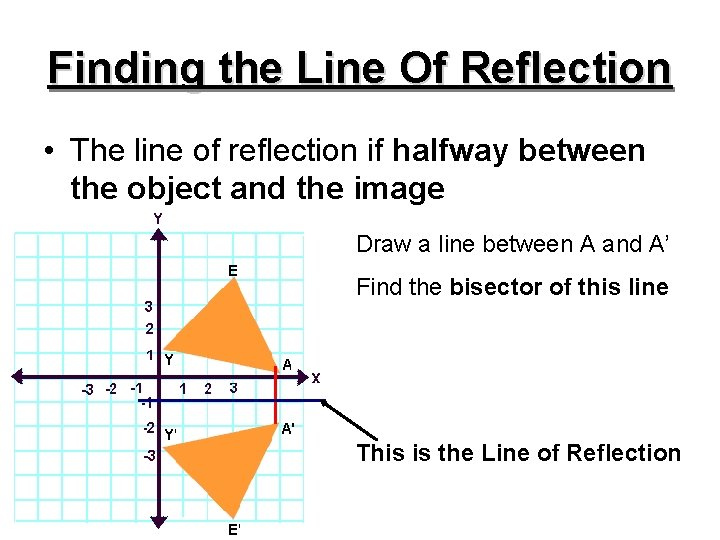Finding the Line Of Reflection • The line of reflection if halfway between the object and the image Draw a line between A and A’ Find the bisector of this line This is the Line of Reflection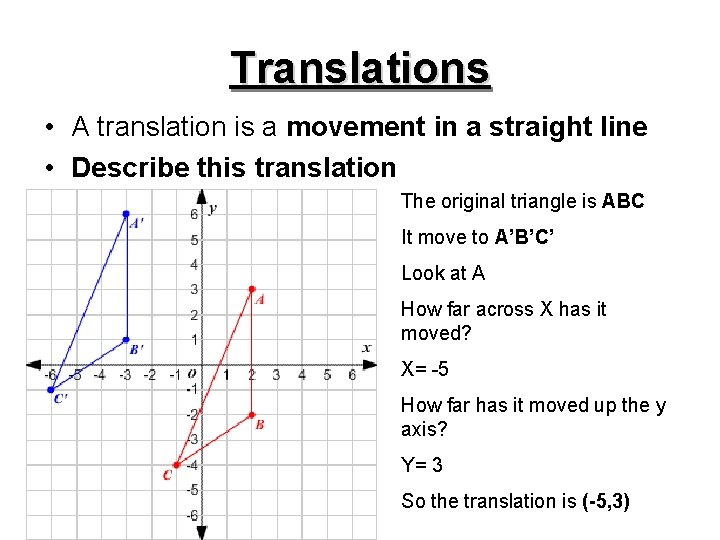Translations • A translation is a movement in a straight line • Describe this translation The original triangle is ABC It move to A’B’C’ Look at A How far across X has it moved? X= -5 How far has it moved up the y axis? Y= 3 So the translation is (-5, 3)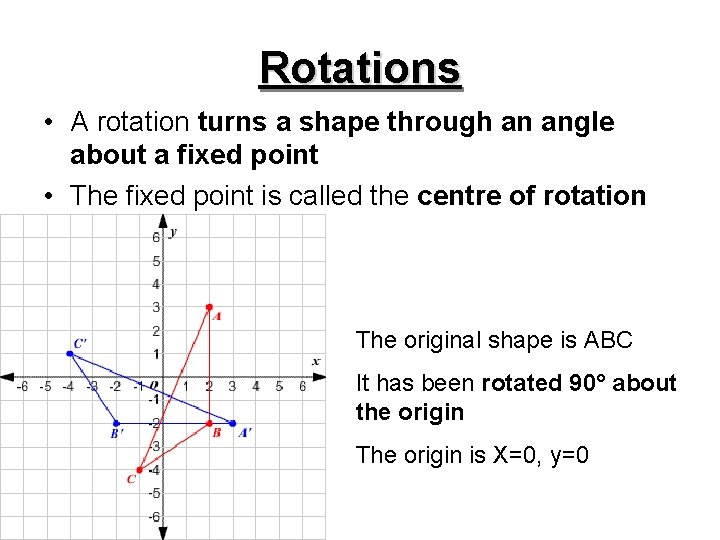Rotations • A rotation turns a shape through an angle about a fixed point • The fixed point is called the centre of rotation The original shape is ABC It has been rotated 90° about the origin The origin is X=0, y=0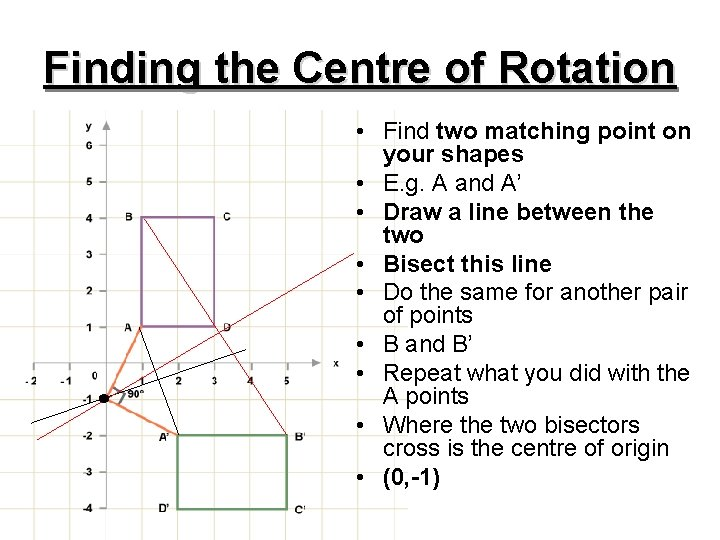Finding the Centre of Rotation • Find two matching point on your shapes • E. g. A and A’ • Draw a line between the two • Bisect this line • Do the same for another pair of points • B and B’ • Repeat what you did with the A points • Where the two bisectors cross is the centre of origin • (0, -1)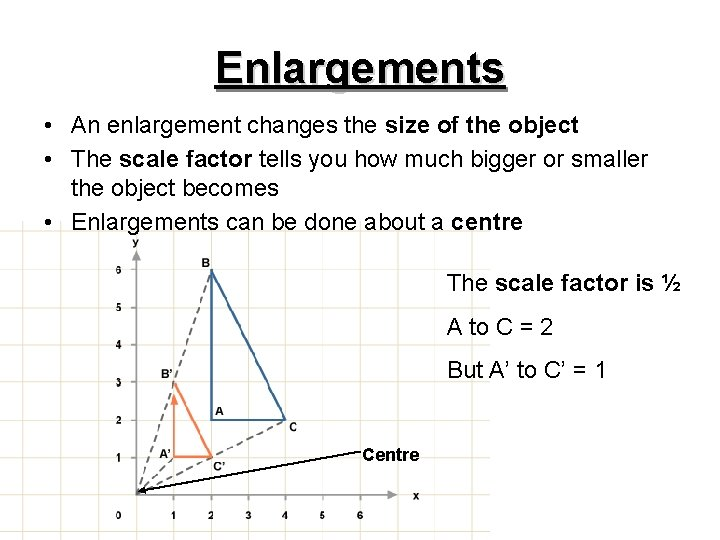Enlargements • An enlargement changes the size of the object • The scale factor tells you how much bigger or smaller the object becomes • Enlargements can be done about a centre The scale factor is ½ A to C = 2 But A’ to C’ = 1 CentreQuestion • Where is the centre and what is the scale factor for this enlargement? The origin is where the black dot is The scale factor is 3 3 9Question • Enlarge triangle ABC by a scale factor of 2 Draw line from O through each of the point A, B and C A’ C’ Double the distance from O to A Plot Point A’ Repeat for B And C B’ Join up these points to make the enlarged shapeSimilar Objects • If two objects have the same shape, but are different sizes they are similar These triangles are similar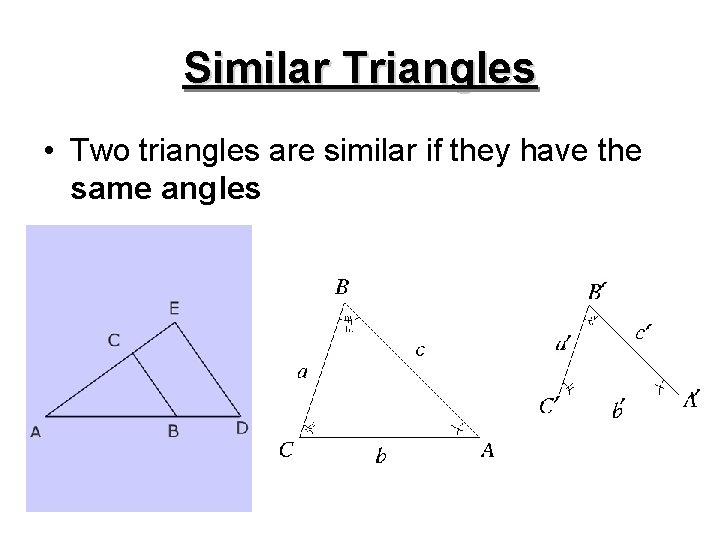Similar Triangles • Two triangles are similar if they have the same anglesCorresponding Angles • On these similar triangles β and Ө angles are the same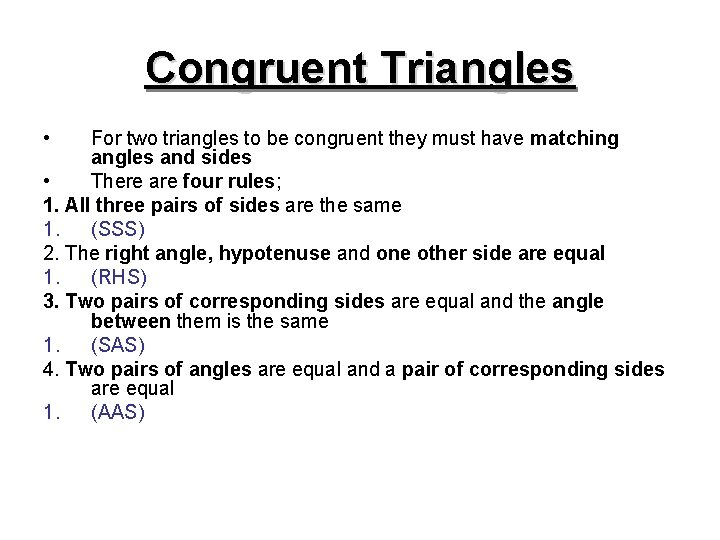Congruent Triangles • For two triangles to be congruent they must have matching angles and sides • There are four rules; 1. All three pairs of sides are the same 1. (SSS) 2. The right angle, hypotenuse and one other side are equal 1. (RHS) 3. Two pairs of corresponding sides are equal and the angle between them is the same 1. (SAS) 4. Two pairs of angles are equal and a pair of corresponding sides are equal 1. (AAS)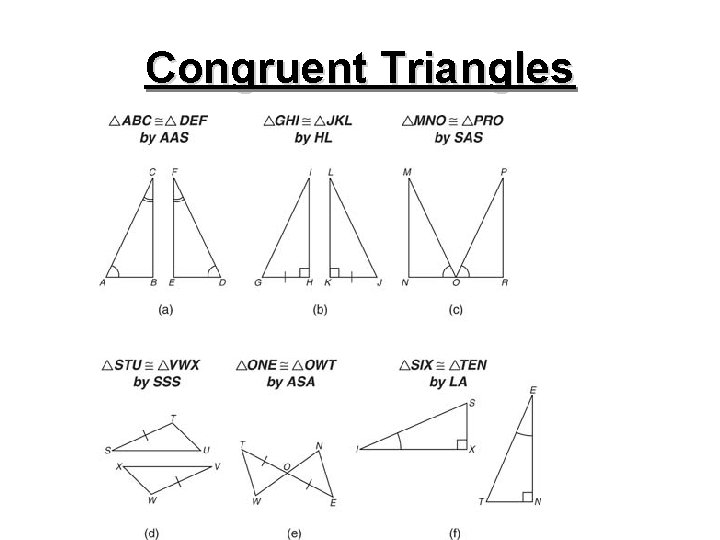Congruent Triangles